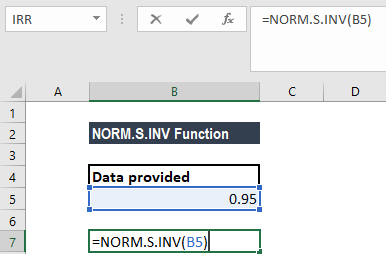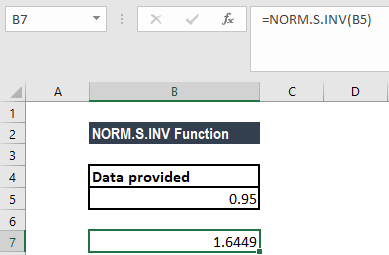# NORM.S.INV Function

Get the inverse of the normal cumulative distribution for a given probability value

## Inverse Normal Cumulative Distribution

The NORM.S.INV Function is categorized under Excel Statistical functions. It will calculate the inverse normal cumulative distribution for a given probability value. Introduced in MS Excel 2010, the NORM.S.INV function is an updated version of the NORMSINV function.

As a financial analyst, the NORM.S.INV is useful in stock market analysis. We can use the function to understand how a portfolio is affected by any additions or withdrawals made.

### NORM.S.INV – A Practical Example

Suppose an appliance manufacturer is investigating the failure times of the heating element of the company’s toasters. The company wishes to determine the time at which specific proportions of the heating elements fail, in order to ideally set the product’s warranty period. Heating element failure times follow a normal distribution, with a mean of 1,500 hours and a standard deviation of 300 hours.

The manufacturer can use the NORM.S.INV function to determine the time by which 5% of the heating elements fail, the times between which 95% of all heating elements fail, and the time at which only 5% of the heating elements continue to function.

If the distribution of the heating element failures follows a normal distribution with a mean of 1,000 and a standard deviation of 300, the time by which 5% of the heating elements are expected to fail is the inverse CDF of 0.05.

### Formula

=NORM.S.INV(probability)

The NORM.S.INV function uses only one argument:

1. Probability (required argument) – This is the probability corresponding to the normal distribution. It is the value at which we want to evaluate the inverse function.

### How to use the NORM.S.INV Function in Excel?

To understand the uses of the NORM.S.INV function, let us consider an example:

#### Example 1 – Inverse normal cumulative distribution

Given a probability of 0.95, to calculate the inverse of the Standard Normal Cumulative Distribution Function, we will use the following formula:We get the result below:### Notes about the Inverse Normal Cumulative Distribution Function

1. #VALUE! error – Occurs if any of the given arguments is non-numeric.
2. #NUM! error – Occurs when the given probability argument is less than zero or greater than one.
3. The precision of NORM.S.INV depends on the precision of NORM.S.DIST. NORM.S.INV uses an iterative search technique.

Thanks for reading CFI’s guide to important Excel functions! By taking the time to learn and master these functions, you’ll significantly speed up your financial analysis. To learn more, check out these additional CFI resources:

• Excel Functions for Finance